## Sum values by group in Excel

Sometimes, you may have the requirement to sum values based on group in a table. For example, I have a list of products with their corresponding amounts in another column, now, I want to get the subtotal amount for each product as below screenshot shown. This tutorial will introduce some formulas for solving this task in Excel.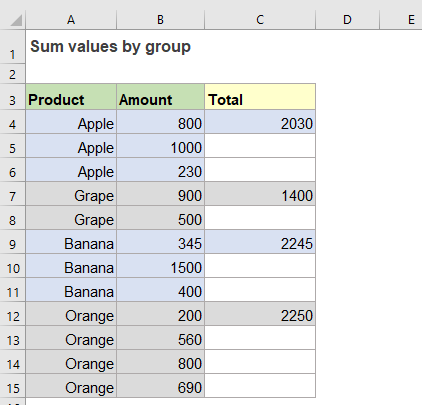#### Sum values by group – subtotals in original data table

Here the generic syntax to sum values based on group in Excel should be like this:

=IF(group_name=cell_above_group_name, “” ,SUMIF(group_range,group_name,sum_range))
• group_name: The cell with the group name you want to sum;
• cell_above_group_name: The cell above the group name;
• group_range: The range of cells contains the group names;
• sum_range: The range of cells corresponding to the specified group name to sum together.

If the data are sorted by the grouping column, the same product is arranged together as below screenshot shown. To subtotal data by group, you can create formulas based on the IF and SUMIF functions.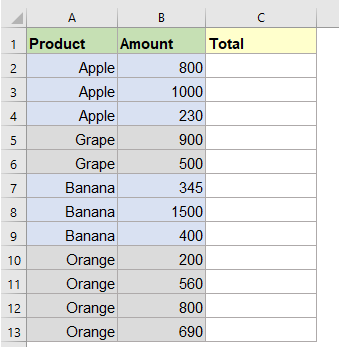1. Please copy and paste the following formula into a blank cell beside your data:

=IF(A2=A1,"",SUMIF(\$A\$2:\$A\$13,A2,\$B\$2:\$B\$13))
• Note: In the formula:
• A1 is the heading cell, and A2 is the first cell which contains the product name you want to use;
• A2:A13 is the list contains the product names you want to sum based on;
• B2:B13 is the column data you want to get the subtotal.

2. And then, drag the fill handle down to the cells that you want to use this formula, and the subtotals are calculated based on each product name, see screenshot: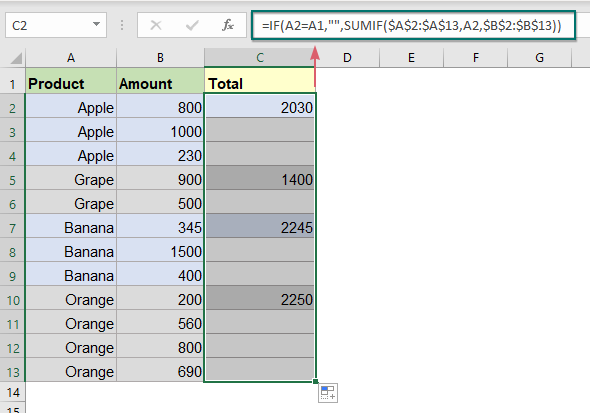##### Explanation of the formula:

=IF(A2=A1,"",SUMIF(\$A\$2:\$A\$13,A2,\$B\$2:\$B\$13))

• SUMIF(\$A\$2:\$A\$13,A2,\$B\$2:\$B\$13): This SUMIF function will sum only the values in range B2:B13 if their corresponding values in range A2:A13 equal to the criteria A2.
• IF(A2=A1,"",SUMIF(\$A\$2:\$A\$13,A2,\$B\$2:\$B\$13)): This IF function checks each value in column A if its value is the same as the value in the above cell. For example, if the cell value A2 is equal to cell A1, nothing ( “” ) will return, if not match, the result of the SUMIF function will be returned.

#### Sum values by group – subtotals in another location

If the values of each group are not arranged together and listed randomly in the column, to sum the matching values based on group or category, you should extract each group name from the product names, and then get the subtotal based on this group name as below screenshot shown.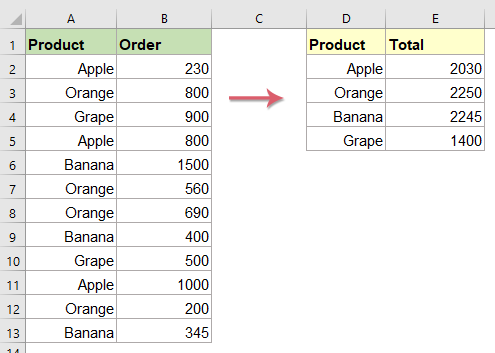1. First, please extract the unique group name by using the following array formula, then press Ctrl + Shift + Enter keys together to get the first result.

=INDEX(\$A\$2:\$A\$13,MATCH(0,COUNTIF(\$D\$1:D1,\$A\$2:\$A\$13),0))
• Note: In the formula:
• A2:A13 is the range of cells where to extract all unique values;
• D1 is the cell above your entered formula.

2. And then, select the formula cell and drag the fill handle down to the cells until all product names are displayed, see screenshot: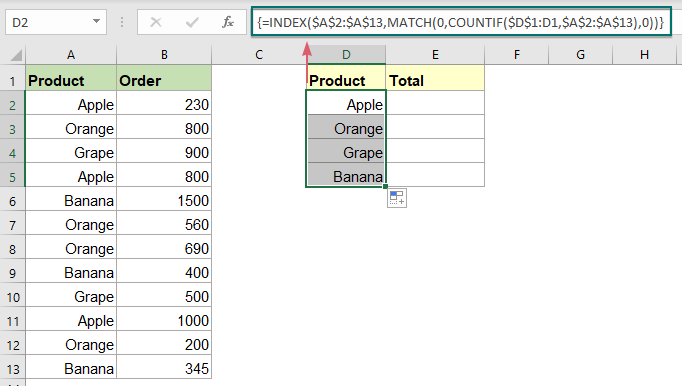3. Now, you can sum the values by the group name you have extracted. In this case, a SUMIF function will be used here, please enter the below formula into a blank cell, in this example, I will put it into cell E2.

=SUMIF(\$A\$2:\$A\$13,D2,\$B\$2:\$B\$13)

4. Then, drag the fill handle down to copy this formula to return the total order of other groups, see screenshot: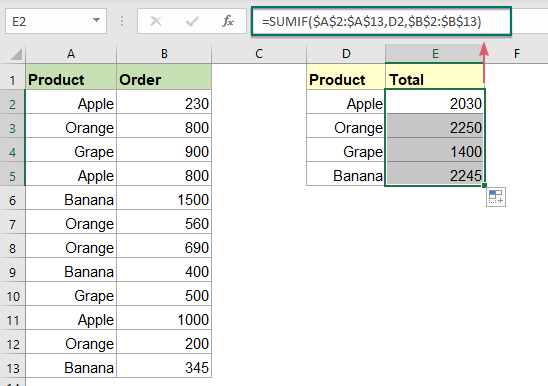#### Relative function used:

• SUMIF:
• The SUMIF function can help to sum cells based on one criterion.
• IF:
• The IF function tests for a specific condition and returns the corresponding value that you supply for TRUE or FALSE.

#### More articles:

• Subtotal Invoice Amounts By Age
• To sum the invoice amounts based on age as below screenshot shown may be a common task in Excel, this tutorial will show how to subtotal invoice amounts by age with a normal SUMIF function.
• Sum Smallest Or Bottom N Values
• In Excel, it is easy for us to sum a range of cells by using the SUM function. Sometimes, you may need to sum the smallest or bottom 3, 5 or n numbers in a data range as below screenshot shown. In this case, the SUMPRODUCT together with the SMALL function can help you to solve this problem in Excel.
• Sum Smallest Or Bottom N Values Based On Criteria
• In previous tutorial, we have discussed how to sum the smallest n values in a data range. In this article, we will perform a further advanced operation – to sum the lowest n values based on one or more criteria in Excel.

### The Best Office Productivity Tools

#### Kutools for Excel - Helps You To Stand Out From Crowd

 Popular Features: Find, Highlight or Identify Duplicates  |  Delete Blank Rows  |  Combine Columns or Cells without Losing Data  |  Round without Formula ... Super VLookup: Multiple Criteria  |  Multiple Value  |  Across Multi-Sheets  |  Fuzzy Lookup... Adv. Drop-down List: Easy Drop Down List  |  Dependent Drop Down List  |  Multi-select Drop Down List... Column Manager: Add a Specific Number of Columns  |  Move Columns  |  Toggle Visibility Status of Hidden Columns  |  Compare Columns to Select Same & Different Cells ... Featured Features: Grid Focus  |  Design View  |  Big Formula Bar  |  Workbook & Sheet Manager | Resource Library (Auto Text)  |  Date Picker  |  Combine Worksheets  |  Encrypt/Decrypt Cells  |  Send Emails by List  |  Super Filter  |  Special Filter (filter bold/italic/strikethrough...) ... Top 15 Toolsets:  12 Text Tools (Add Text, Remove Characters ...)  |  50+ Chart Types (Gantt Chart ...)  |  40+ Practical Formulas (Calculate age based on birthday ...)  |  19 Insertion Tools (Insert QR Code, Insert Picture from Path ...)  |  12 Conversion Tools (Numbers to Words, Currency Conversion ...)  |  7 Merge & Split Tools (Advanced Combine Rows, Split Excel Cells ...)  |  ... and more

Kutools for Excel Boasts Over 300 Features, Ensuring That What You Need is Just A Click Away...#### Office Tab - Enable Tabbed Reading and Editing in Microsoft Office (include Excel)

• One second to switch between dozens of open documents!
• Reduce hundreds of mouse clicks for you every day, say goodbye to mouse hand.
• Increases your productivity by 50% when viewing and editing multiple documents.
• Brings Efficient Tabs to Office (include Excel), Just Like Chrome, Edge and Firefox.